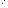# How To Name Simple Alkene Chains

An alkene is a molecule made up entirely of carbon and hydrogen where one or more carbon atoms are connected by double bonds. The general formula for an alkene is CnH2n where n is the number of carbon atoms in the molecule.
Alkenes are named by adding the -ene suffix to the prefix associated with the number of carbon atoms present in the molecule. A number and dash before the name denote the number of the carbon atom in the chain that begins the double bond.
For example, 1-hexene is a six carbon chain where the double bond is between the first and second carbon atoms.
Click image to enlarge the molecule.

## Ethene

Number of Carbons: 2
Prefix: eth- Number of Hydrogens: 2(2) = 4
Molecular Formula: C2H4

## Propene

Number of Carbons: 3
Prefix: prop- Number of Hydrogens: 2(3)= 6
Molecular Formula: C3H6

## Butene

Number of Carbons: 4
Prefix: but- Number of Hydrogens: 2(4) = 8
Molecular Formula: C4H8

## Pentene

Number of Carbons: 5
Prefix: pent- Number of Hydrogens: 2(5) = 10
Molecular Formula: C5H10

## Hexene

Number of Carbons: 6
Prefix: hex- Number of Hydrogens: 2(6)= 12
Molecular Formula: C6H12

## Heptene

Number of Carbons: 7
Prefix: hept- Number of Hydrogens: 2(7) = 14
Molecular Formula: C7H14

## Octene

Number of Carbons: 8
Prefix: oct- Number of Hydrogens: 2(8) = 16
Molecular Formula: C8H16

## Nonene

Number of Carbons: 9
Prefix: non- Number of Hydrogens: 2(9) = 18
Molecular Formula: C9H18

## Decene

Number of Carbons: 10
Prefix: dec- Number of Hydrogens: 2(10) = 20
Molecular Formula: C10H20

## Isomer Numbering SchemeThis shows three isomers of the hexene alkene molecule: 1-hexene, 2-hexene and 3-hexene. The carbons are numbered from left to right to show the location of the carbon double bonds.  ThoughtCo

These three structures illustrate the numbering scheme for isomers of alkene chains. The carbon atoms are numbered from left to right. The number represents the location of the first carbon atom that is part of the double bond.
In this example: 1-hexene has the double bond between carbon 1 and carbon 2, 2-hexene between carbon 2 and 3, and 3-hexene between carbon 3 and carbon 4.
4-hexene is identical to 2-hexene and 5-hexene is identical to 1-hexene. In these cases, the carbon atoms would be numbered from right to left so the lowest number would be used to represent the molecule's name.

Format
mla apa chicago